Contrast tests with ART

Introduction

The aligned-rank transform (ART) allows for non-parametric analyses of variance (Wobbrock et al. 2011). But how should we do contrast tests with ART?

Contrasts involving levels of single factors, combinations of factors, or differences of differences across two factors can be performed by conducting those contrasts on a linear model aligned-and-ranked on the factors involved in the contrasts. This linear model may be one of the models used in the ART procedure, or it may require concatenating factors and constructing a new model, a procedure called ART contrasts or ART-C (Elkin et al. 2021).

The art.con() function selects the appropriate model given a desired set of contrasts and then performs the requested contrasts. This page explains when and why a separate aligning-and-ranking procedure is needed to conduct contrasts and demonstrates how to conduct those contrasts using the art.con() function within the ART paradigm.

If you are not sure when/how to select the appropriate aligned-and-ranked linear model for a given contrast (i.e. when to use ART versus ART-C), the art.con() function demonstrated in this vignette will select the appropriate method given a contrast specification.

Contents

1. Test Dataset: Description of the test data we will use to compare a linear model against ART
2. Contrast tests of main effects: Demo of conducting contrasts within a single factor (no interaction)
3. Tests of differences in pairwise combinations of levels between factors in interactions: This type of interaction contrast must be conducted with ART-C
4. Tests of differences of differences in interactions: This type of interaction contrast can be conducted with ART

Libraries needed for this vignette

library(dplyr)      #data_frame, %>%, filter, summarise, group_by
library(emmeans)    #emmeans, contrast
library(phia)       #testInteractions
library(ARTool)     #art, artlm
library(ggplot2)    #ggplot, stat_..., geom_..., etc

Test dataset

Let’s generate some test data where we actually know what the effects are. Specifically,

n_per_group = 150
df = tibble(
X1 = factor(c(rep("A", n_per_group), rep("B", n_per_group))),
X2 = factor(rep(c("C","D","E"), n_per_group * 2/3)),
Y = rnorm(
n_per_group * 2,
(X1 == "B")
+ 2* (X2 == "D")
+ 2 * (X1 == "B" & X2 == "D")
- 2 * (X1 == "A" & X2 == "D")
+ 2 * (X2 == "E")
)
)

This is normally-distributed error with the same variance at all levels, so we can compare the results of ART and ART-C to a linear model, which will correctly estimate the effects.

I pre-ran the above code and saved it as InteractionTestData so that the text here is consistent:

data(InteractionTestData, package = "ARTool")
df = InteractionTestData    #save some typing

The “true” means from the model look like this:

X1 X2 Mean
A C or D 0
A E 2
B C 1
B D 5
B E 3

Which we can see pretty well:

# variant of the Dark2 colorbrewer scale with specific name mappings (so
# we can keep color -> name mapping consistent throughout this document)
palette = c("#1b9e77", "#d95f02", "#7570b3")
names(palette) = c("C", "D", "E")

df %>%
ggplot(aes(x = X1, y = Y, color = X2)) +
geom_violin(trim = FALSE, adjust = 1.5) +
geom_point(pch = "-", size = 4) +
stat_summary(fun = mean, geom = "point", size = 4) +
stat_summary(aes(group = X2), fun = mean, geom = "line", size = 1) +
stat_summary(aes(x = 1.5, group = NA), fun = mean, geom = "point", size = 9, pch = "+") +
scale_y_continuous(breaks = seq(-6, 10, by = 2), minor_breaks = -6:10) +
scale_color_manual(guide = FALSE, values = palette) +
coord_cartesian(ylim = c(-6, 10)) +
facet_grid(. ~ X2)
## Warning: It is deprecated to specify guide = FALSE to remove a guide. Please
## use guide = "none" instead.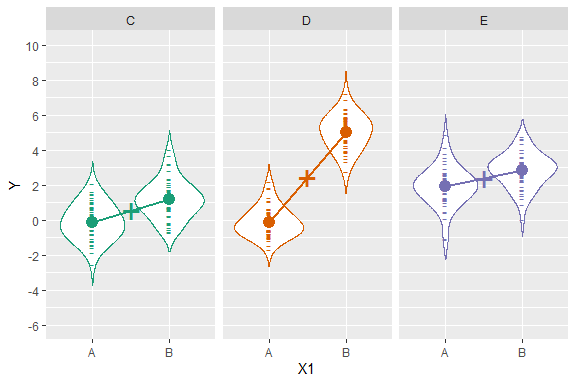And “true” means for each level (averaging over the levels of the other factor):

Level Mean
X1 == A 0.66666
X1 == B 3
X2 == C 0.5
X2 == D 2.5
X2 == E 2.5

Let’s fit a linear model:

m.linear = lm(Y ~ X1*X2, data = df)
anova(m.linear)
## Analysis of Variance Table
##
## Response: Y
##            Df Sum Sq Mean Sq F value    Pr(>F)
## X1          1 445.50  445.50  439.44 < 2.2e-16 ***
## X2          2 236.44  118.22  116.61 < 2.2e-16 ***
## X1:X2       2 270.40  135.20  133.36 < 2.2e-16 ***
## Residuals 294 298.06    1.01
## ---
## Signif. codes:  0 '***' 0.001 '**' 0.01 '*' 0.05 '.' 0.1 ' ' 1

Now with ART:

m.art = art(Y ~ X1*X2, data = df)
anova(m.art)
## Analysis of Variance of Aligned Rank Transformed Data
##
## Table Type: Anova Table (Type III tests)
## Model: No Repeated Measures (lm)
## Response: art(Y)
##
##         Df Df.res F value     Pr(>F)
## 1 X1     1    294  488.33 < 2.22e-16 ***
## 2 X2     2    294  114.35 < 2.22e-16 ***
## 3 X1:X2  2    294  145.65 < 2.22e-16 ***
## ---
## Signif. codes:   0 '***' 0.001 '**' 0.01 '*' 0.05 '.' 0.1 ' ' 1

Both have significance at all levels (expected given the number of samples and the “true” effects) and similar enough F values. The real question is whether/what kind of contrast tests make sense.

Contrast tests of main effects

For the main effects, let’s look at contrast tests for the linear model:

contrast(emmeans(m.linear, ~ X1), method = "pairwise")
##  contrast estimate    SE  df t.ratio p.value
##  A - B       -2.44 0.116 294 -20.963  <.0001
##
## Results are averaged over the levels of: X2
contrast(emmeans(m.linear, ~ X2), method = "pairwise")
##  contrast estimate    SE  df t.ratio p.value
##  C - D     -1.9121 0.142 294 -13.428  <.0001
##  C - E     -1.8530 0.142 294 -13.013  <.0001
##  D - E      0.0592 0.142 294   0.415  0.9093
##
## Results are averaged over the levels of: X1
## P value adjustment: tukey method for comparing a family of 3 estimates

These are about right: The “true” effect for A - B is -2.3333, for C - D and C - E is -2, and for D - E is 0 (see table above). For ART, artlm() will return the appropriate linear model for single-factor contrasts, which we can then use with a library that does contrasts (such as emmeans()):

# this works for single factors, though it is better (more general) to use
# artlm.con() or art.con() (see below)
contrast(emmeans(artlm(m.art, "X1"), ~ X1), method = "pairwise")
##  contrast estimate   SE  df t.ratio p.value
##  A - B        -137 6.19 294 -22.098  <.0001
##
## Results are averaged over the levels of: X2
contrast(emmeans(artlm(m.art, "X2"), ~ X2), method = "pairwise")
##  contrast estimate   SE  df t.ratio p.value
##  C - D     -123.13 9.28 294 -13.272  <.0001
##  C - E     -119.81 9.28 294 -12.914  <.0001
##  D - E        3.32 9.28 294   0.358  0.9319
##
## Results are averaged over the levels of: X1
## P value adjustment: tukey method for comparing a family of 3 estimates

This is about right (effects in the same direction, the estimates aren’t the same because they are on the scale of ranks and not the data, but the t values are similar to the linear model, as we should hope). However, I recommend using artlm.con() instead of artlm(), as it will also return the correct model in this case but not in the general case, as we will see below. Using artlm.con(), we get the same result as before:

contrast(emmeans(artlm.con(m.art, "X1"), ~ X1), method = "pairwise")
##  contrast estimate   SE  df t.ratio p.value
##  A - B        -137 6.19 294 -22.098  <.0001
##
## Results are averaged over the levels of: X2
contrast(emmeans(artlm.con(m.art, "X2"), ~ X2), method = "pairwise")
##  contrast estimate   SE  df t.ratio p.value
##  C - D     -123.13 9.28 294 -13.272  <.0001
##  C - E     -119.81 9.28 294 -12.914  <.0001
##  D - E        3.32 9.28 294   0.358  0.9319
##
## Results are averaged over the levels of: X1
## P value adjustment: tukey method for comparing a family of 3 estimates

We can also use the shortcut function art.con(), which will perform the appropriate call to both artlm.con() and emmeans() for the desired contrast:

art.con(m.art, "X1")
##  contrast estimate   SE  df t.ratio p.value
##  A - B        -137 6.19 294 -22.098  <.0001
##
## Results are averaged over the levels of: X2
art.con(m.art, "X2")
##  contrast estimate   SE  df t.ratio p.value
##  C - D     -123.13 9.28 294 -13.272  <.0001
##  C - E     -119.81 9.28 294 -12.914  <.0001
##  D - E        3.32 9.28 294   0.358  0.9319
##
## Results are averaged over the levels of: X1
## P value adjustment: tukey method for comparing a family of 3 estimates

Within a single factor ART (i.e., artlm()) and ART-C (artlm.con()) are mathematically equivalent, so the contrast tests for ART and ART-C have the same results.

Tests of differences in pairwise combinations of levels between factors in interactions

Now let’s look at tests of differences in combinations of levels between factors:

contrast(emmeans(m.linear, ~ X1:X2), method = "pairwise")
##  contrast   estimate    SE  df t.ratio p.value
##  A C - B C -1.290161 0.201 294  -6.407  <.0001
##  A C - A D -0.000506 0.201 294  -0.003  1.0000
##  A C - B D -5.113912 0.201 294 -25.395  <.0001
##  A C - A E -2.044007 0.201 294 -10.150  <.0001
##  A C - B E -2.952089 0.201 294 -14.660  <.0001
##  B C - A D  1.289654 0.201 294   6.404  <.0001
##  B C - B D -3.823751 0.201 294 -18.988  <.0001
##  B C - A E -0.753846 0.201 294  -3.743  0.0030
##  B C - B E -1.661928 0.201 294  -8.253  <.0001
##  A D - B D -5.113406 0.201 294 -25.392  <.0001
##  A D - A E -2.043501 0.201 294 -10.148  <.0001
##  A D - B E -2.951583 0.201 294 -14.657  <.0001
##  B D - A E  3.069905 0.201 294  15.245  <.0001
##  B D - B E  2.161823 0.201 294  10.735  <.0001
##  A E - B E -0.908082 0.201 294  -4.509  0.0001
##
## P value adjustment: tukey method for comparing a family of 6 estimates

If we naively apply the ART procedure (using artlm()), we will get incorrect results:

#DO NOT DO THIS!
contrast(emmeans(artlm(m.art, "X1:X2"), ~ X1:X2), method = "pairwise")
##  contrast  estimate   SE  df t.ratio p.value
##  A C - B C     76.9 12.4 294   6.202  <.0001
##  A C - A D    125.1 12.4 294  10.091  <.0001
##  A C - B D    -45.3 12.4 294  -3.650  0.0042
##  A C - A E    -12.1 12.4 294  -0.974  0.9258
##  A C - B E     87.2 12.4 294   7.030  <.0001
##  B C - A D     48.2 12.4 294   3.889  0.0017
##  B C - B D   -122.2 12.4 294  -9.853  <.0001
##  B C - A E    -89.0 12.4 294  -7.177  <.0001
##  B C - B E     10.3 12.4 294   0.828  0.9623
##  A D - B D   -170.4 12.4 294 -13.742  <.0001
##  A D - A E   -137.2 12.4 294 -11.066  <.0001
##  A D - B E    -38.0 12.4 294  -3.062  0.0288
##  B D - A E     33.2 12.4 294   2.676  0.0832
##  B D - B E    132.4 12.4 294  10.680  <.0001
##  A E - B E     99.2 12.4 294   8.004  <.0001
##
## P value adjustment: tukey method for comparing a family of 6 estimates

Compare these to the linear model: very different results!

The linear model tests are easy to interpret: they tell us the expected mean difference between combinations of levels.

The ART results are more difficult to interpret. Take A,C - A,D, which looks like this:

df %>%
filter(X1 == "A", X2 %in% c("C", "D")) %>%
ggplot(aes(x = X1:X2, y = Y, color = X2)) +
geom_violin(trim = FALSE, adjust = 1.5) +
geom_point(pch = "-", size = 4) +
stat_summary(fun = mean, geom = "point", size = 4) +
scale_y_continuous(breaks = seq(-6, 10, by = 2), minor_breaks = -6:10) +
scale_color_manual(guide = FALSE, values = palette) +
coord_cartesian(ylim=c(-6,10))
## Warning: It is deprecated to specify guide = FALSE to remove a guide. Please
## use guide = "none" instead.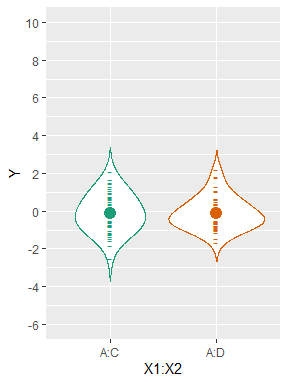The linear model correctly estimates this difference as approximately 0, which is both the true effect and what we should expect from a visual inspection of the data. Unlike the linear model, the ART model gives us a statistically significant difference between A,C and A,D, which if we interpret in the same way as the linear model is obviously incorrect.

The key here is to understand that ART is reporting differences with the main effects subtracted out. That is, the A,C - A,D effect is something like the difference between this combination of levels if we first subtracted out the effect of C - D. We can see this if we take the ART estimate for C - D in the emmeans output for X2 above (-123.13) and the ART estimate for A,C - A,D (125.12) here, we can get approximate an estimate of the difference (-123.13 + 125.12 == 1.99) that is consistent with the expected 0 (given the SE here).

The ART-C procedure was developed to align and rank data specifically for contrasts involving levels from any number of factors, and is available through art.con():

art.con(m.art, "X1:X2")
##  contrast  estimate   SE  df t.ratio p.value
##  A,C - A,D     1.96 8.91 294   0.220  0.9999
##  A,C - A,E  -100.96 8.91 294 -11.333  <.0001
##  A,C - B,C   -63.16 8.91 294  -7.090  <.0001
##  A,C - B,D  -205.76 8.91 294 -23.096  <.0001
##  A,C - B,E  -141.84 8.91 294 -15.921  <.0001
##  A,D - A,E  -102.92 8.91 294 -11.553  <.0001
##  A,D - B,C   -65.12 8.91 294  -7.310  <.0001
##  A,D - B,D  -207.72 8.91 294 -23.316  <.0001
##  A,D - B,E  -143.80 8.91 294 -16.141  <.0001
##  A,E - B,C    37.80 8.91 294   4.243  0.0004
##  A,E - B,D  -104.80 8.91 294 -11.764  <.0001
##  A,E - B,E   -40.88 8.91 294  -4.589  0.0001
##  B,C - B,D  -142.60 8.91 294 -16.007  <.0001
##  B,C - B,E   -78.68 8.91 294  -8.832  <.0001
##  B,D - B,E    63.92 8.91 294   7.175  <.0001
##
## P value adjustment: tukey method for comparing a family of 6 estimates

Like the linear model, art.con() correctly estimates the difference between A,C - A,D as approximately 0. In fact, its results agree with the linear model for all contrasts conducted. (Note that the art.con() and linear model results appear in a different order).

The syntax used above is consistent with term syntax used by artlm(). art.con() also accepts the formula syntax accepted by emmeans::emmeans(). We can conduct the same contrasts as above using the following syntax:

art.con(m.art, ~ X1*X2)
##  contrast  estimate   SE  df t.ratio p.value
##  A,C - A,D     1.96 8.91 294   0.220  0.9999
##  A,C - A,E  -100.96 8.91 294 -11.333  <.0001
##  A,C - B,C   -63.16 8.91 294  -7.090  <.0001
##  A,C - B,D  -205.76 8.91 294 -23.096  <.0001
##  A,C - B,E  -141.84 8.91 294 -15.921  <.0001
##  A,D - A,E  -102.92 8.91 294 -11.553  <.0001
##  A,D - B,C   -65.12 8.91 294  -7.310  <.0001
##  A,D - B,D  -207.72 8.91 294 -23.316  <.0001
##  A,D - B,E  -143.80 8.91 294 -16.141  <.0001
##  A,E - B,C    37.80 8.91 294   4.243  0.0004
##  A,E - B,D  -104.80 8.91 294 -11.764  <.0001
##  A,E - B,E   -40.88 8.91 294  -4.589  0.0001
##  B,C - B,D  -142.60 8.91 294 -16.007  <.0001
##  B,C - B,E   -78.68 8.91 294  -8.832  <.0001
##  B,D - B,E    63.92 8.91 294   7.175  <.0001
##
## P value adjustment: tukey method for comparing a family of 6 estimates

We can also manually conduct the contrasts with emmeans::emmeans() (or another library for running contrasts) by first extracting the linear model with artlm.con(). Note that the contrasts must be performed on the variable constructed by artlm.con() with the names of the factors involved concatenated together (X1X2):

contrast(emmeans(artlm.con(m.art, "X1:X2"), ~ X1X2), method = "pairwise")
##  contrast  estimate   SE  df t.ratio p.value
##  A,C - A,D     1.96 8.91 294   0.220  0.9999
##  A,C - A,E  -100.96 8.91 294 -11.333  <.0001
##  A,C - B,C   -63.16 8.91 294  -7.090  <.0001
##  A,C - B,D  -205.76 8.91 294 -23.096  <.0001
##  A,C - B,E  -141.84 8.91 294 -15.921  <.0001
##  A,D - A,E  -102.92 8.91 294 -11.553  <.0001
##  A,D - B,C   -65.12 8.91 294  -7.310  <.0001
##  A,D - B,D  -207.72 8.91 294 -23.316  <.0001
##  A,D - B,E  -143.80 8.91 294 -16.141  <.0001
##  A,E - B,C    37.80 8.91 294   4.243  0.0004
##  A,E - B,D  -104.80 8.91 294 -11.764  <.0001
##  A,E - B,E   -40.88 8.91 294  -4.589  0.0001
##  B,C - B,D  -142.60 8.91 294 -16.007  <.0001
##  B,C - B,E   -78.68 8.91 294  -8.832  <.0001
##  B,D - B,E    63.92 8.91 294   7.175  <.0001
##
## P value adjustment: tukey method for comparing a family of 6 estimates

Tests of differences of differences in interactions

You may also with to test differences of differences; e.g., for the interaction X1:X2, we might ask, is the difference A - B different when X2 = C compared to when X2 = D?. We can test this using the interaction argument to art.con(). When the interaction argument is supplied to art.con, differences of differences are tested on data that has been aligned-and-ranked using the original ART method (i.e., the data is not aligned-and-ranked using the ART-C method, as it is not necessary for these contrasts).

Before we test, let’s try to visualize what’s going on in just this interaction:

plot_interaction_for_X2_levels = function(...) {
x2_levels = c(...)
df. = filter(df, X2 %in% x2_levels)

X1_in_X2 = df. %>%
group_by(X1, X2) %>%
summarise(Y = mean(Y), .groups = "drop") %>%

print(
ggplot(df., aes(x = X1, y = Y, color = X2)) +
geom_violin(trim = FALSE, adjust = 1.5) +
geom_point(pch = "-", size = 4) +
stat_summary(fun = mean, geom = "point", size = 4) +
stat_summary(aes(group = X2), fun = mean, geom = "line", size = 1, linetype = "dashed") +
geom_errorbar(
aes(x = 2.2, ymin = A, ymax = B, y = NULL),
data = X1_in_X2, width = .19, size = 0.8, color = "black"
) +
geom_text(
aes(x = 2.35, y = (A + B)/2, label = paste("A - B |", X2)),
data = X1_in_X2, hjust = 0, size = 5, color = "black"
) +
scale_y_continuous(breaks = seq(-6, 10, by = 2), minor_breaks = -6:10) +
scale_color_manual(guide = FALSE, values = palette[x2_levels]) +
coord_cartesian(xlim = c(0, 3.5), ylim = c(-6,10)) +
facet_grid(. ~ X2)
)
}
plot_interaction_for_X2_levels("C", "D")
## Warning: It is deprecated to specify guide = FALSE to remove a guide. Please
## use guide = "none" instead.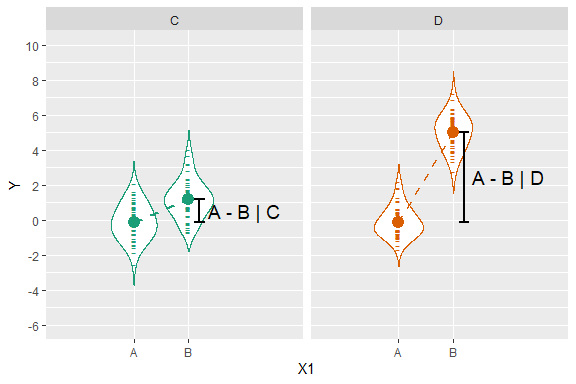The true effect for A - B | C is -1, for A - B | D is -5, and for (A - B | C) - (A - B | D) is (-1) - (-5) = 4. Visually, we’re asking if the two dashed lines in the above plot are parallel. Equivalently, we’re asking if the vertical distance from the mean of A to the mean of B in the left panel (when X2 == C) is the same as the vertical distance between A and B in the right panel (when X2 == D). The true difference between these vertical distances (the “difference of a difference”) is 4, which is also about what we would estimate it to be by looking at the above plot.

We can get the estimate of this “difference of a difference” from the linear model by adding interaction = TRUE to the same call to contrast we made previously:

contrast(emmeans(m.linear, ~ X1:X2), method = "pairwise", interaction = TRUE)
##  X1_pairwise X2_pairwise estimate    SE  df t.ratio p.value
##  A - B       C - D          3.823 0.285 294  13.425  <.0001
##  A - B       C - E         -0.382 0.285 294  -1.342  0.1808
##  A - B       D - E         -4.205 0.285 294 -14.766  <.0001

Here we can interpret the row A - B C - D as the difference between (A - B | C) and (A - B | D), which is estimated as 3.82 (close to the true effect of 4, see the plot above).

We can look at a similar plot for the row A - B C - E:

plot_interaction_for_X2_levels("C", "E")
## Warning: It is deprecated to specify guide = FALSE to remove a guide. Please
## use guide = "none" instead.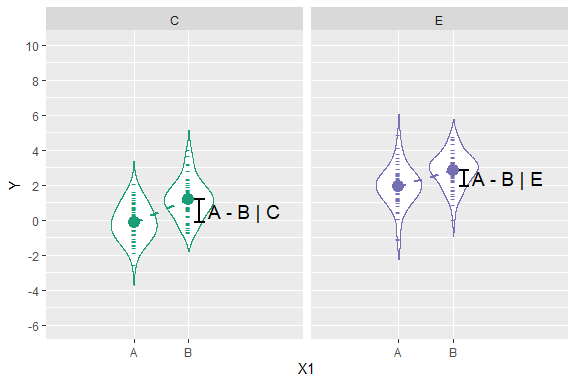Here the true effect for A - B | C is -1, A - B | E is also -1, and (A - B | C) - (A - B | E) is 0. Visually, this sample looks close to the true effects (the height of A - B | C is about the same as A - B | E). From the the row A-B : C-E above we can see that the estimate from the linear model is ~0, as we should hope.

A similar visual analysis finds the estimate for row A - B D - E (~ -4.2) also to be correct (true effect is -4):

plot_interaction_for_X2_levels("D", "E")
## Warning: It is deprecated to specify guide = FALSE to remove a guide. Please
## use guide = "none" instead.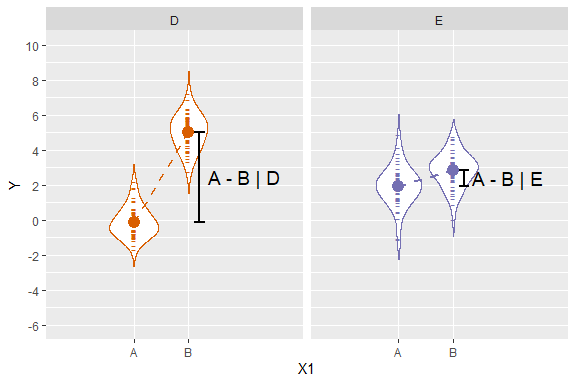Now we look at these differences of differences in ART, using art.con():

art.con(m.art, "X1:X2", interaction = TRUE)
##  X1_pairwise X2_pairwise estimate   SE  df t.ratio p.value
##  A - B       C - D          247.3 17.5 294  14.103  <.0001
##  A - B       C - E          -22.3 17.5 294  -1.274  0.2036
##  A - B       D - E         -269.6 17.5 294 -15.377  <.0001

This is equivalent to:

contrast(emmeans(artlm(m.art, "X1:X2"), ~ X1:X2), method = "pairwise", interaction = TRUE)
##  X1_pairwise X2_pairwise estimate   SE  df t.ratio p.value
##  A - B       C - D          247.3 17.5 294  14.103  <.0001
##  A - B       C - E          -22.3 17.5 294  -1.274  0.2036
##  A - B       D - E         -269.6 17.5 294 -15.377  <.0001

And we see t values consistent with the linear model, and consistent estimates (given the standard error). These types of comparisons work under ART because they do not involve coefficients of main effects (see the description of these tests in vignette("phia")), thus are consistent even when ART has stripped out the main effects.

If you prefer the phia package, the code to run the equivalent tests using the testInteractions function in phia instead of using emmeans is:

testInteractions(artlm(m.art, "X1:X2"), pairwise = c("X1","X2"))
## F Test:
While emmeans() uses t tests in this case, testInteractions() gives the result of equivalent F tests with one numerator degree of freedom (an F test with $$F(1,\nu) = f$$ is equivalent to a two-sided t test with $$t(\nu) = \sqrt{f}$$). I prefer the t test in this case because the t value preserves the direction of the effect (its sign) and is more amenable to calculating interpretable (ish) effect sizes like Cohen’s d. For an example of the latter, see vignette(“art-effect-size”).